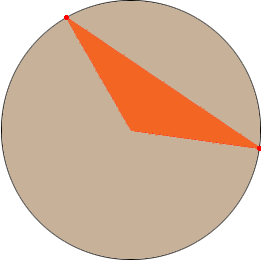Name: Bob Who is asking: Student Level of the question: Secondary Question: Is there any way to obtain the third side of a triangle when 2 sides are the same length (2.18 inches). I also need to find the angles. Thank you Hi Bob, No. Draw a circle of radius 2.18 inches and then draw and two radii of the circle. Join the ends of the radii to form a triangle.What you have is a triangle with two sides of length 2.18 inches and the third side can have any length that is larger than zero and smaller than twice the radius. Penny